Question

A continuous random variable X has probability density function

f(x) = a for −2 < x < 0

bx for 0 < x ≤ 1

0 otherwise

where a and b are constants. It is known that E(X) = 0.

(a) Determine a and b.

(b) Find Var(X)

(c) Find the median of X, i.e. a number m such that P(X ≤ m) = 1/2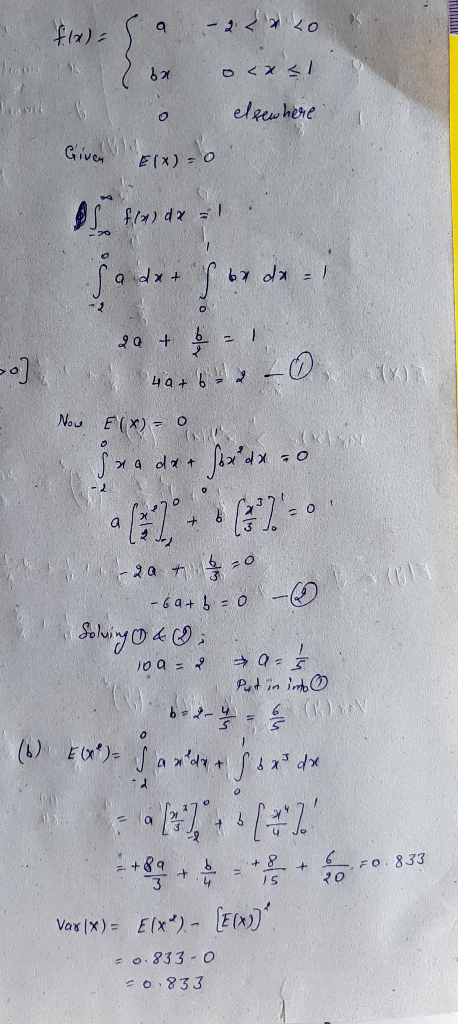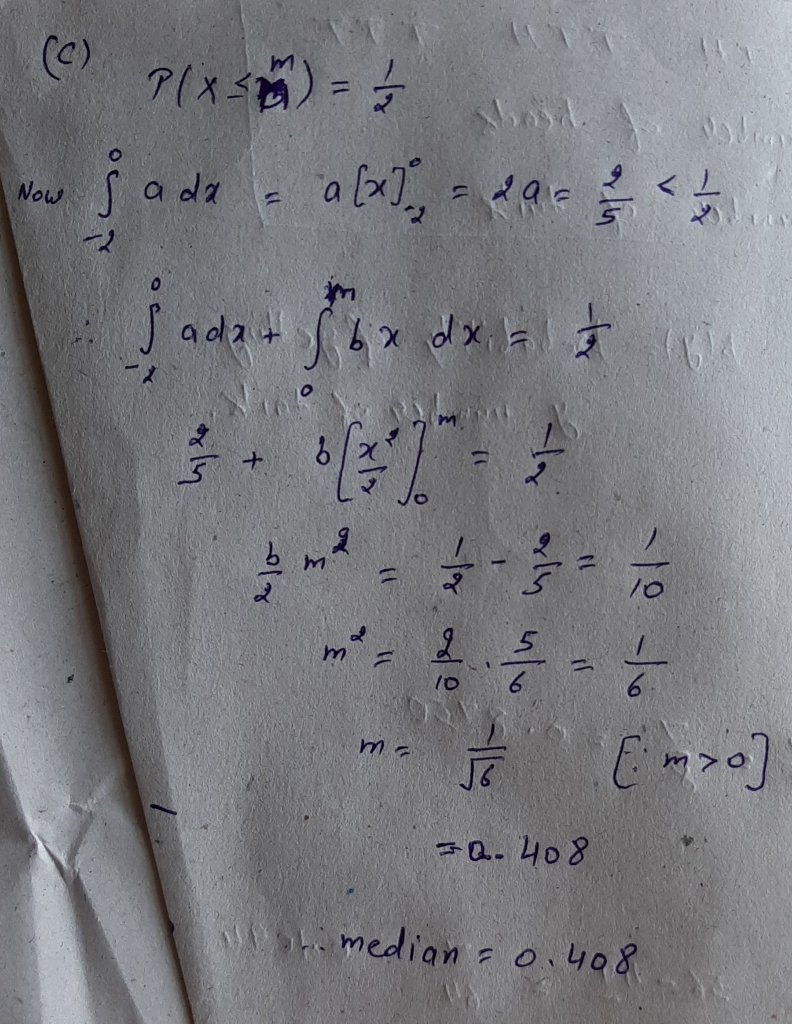#### Earn Coins

Coins can be redeemed for fabulous gifts.

Similar Homework Help Questions
• ### 3. Let X be a continuous random variable with probability density function ax2 + bx f(0)...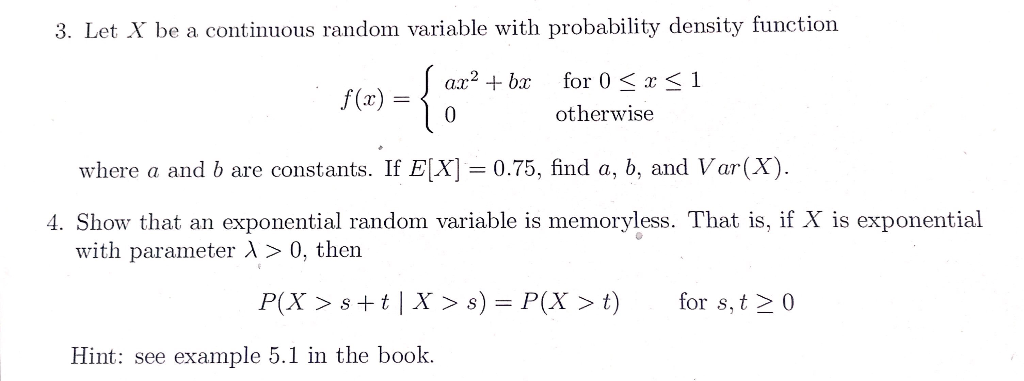3. Let X be a continuous random variable with probability density function ax2 + bx f(0) = -{ { for 0 < x <1 otherwise 0 where a and b are constants. If E(X) = 0.75, find a, b, and Var(X). 4. Show that an exponential random variable is memoryless. That is, if X is exponential with parameter > 0, then P(X > s+t | X > s) = P(X > t) for s,t> 0 Hint: see example 5.1 in...

• ### 1. Let X be a continuous random variable with probability density function f(x) = { if...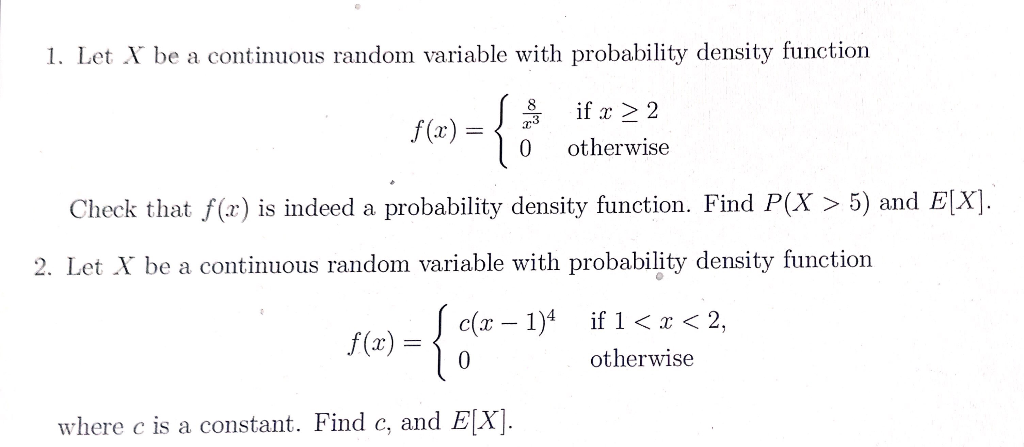1. Let X be a continuous random variable with probability density function f(x) = { if x > 2 otherwise 0 Check that f(-x) is indeed a probability density function. Find P(X > 5) and E[X]. 2. Let X be a continuous random variable with probability density function f(x) = = { SE otherwise where c is a constant. Find c, and E[X].

• ### 5. (20%) Let X be a continuous random variable whose probability density function is fr(x) (a +bx)%0(x) (a) If Ex)f...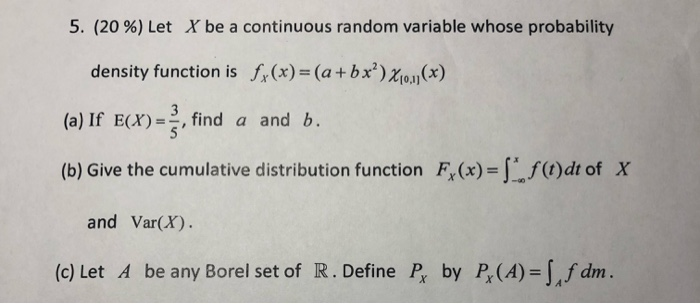5. (20%) Let X be a continuous random variable whose probability density function is fr(x) (a +bx)%0(x) (a) If Ex)f find a and b. (b) Give the cumulative distribution function F,(x) f()dt of X and Var(X) (c) Let A be any Borel set of R. Define P by P(A) [,f dm 5. (20%) Let X be a continuous random variable whose probability density function is fr(x) (a +bx)%0(x) (a) If Ex)f find a and b. (b) Give the cumulative distribution...

• ### b. Let X be a continuous random variable with probability density function f(x) = kx2 if...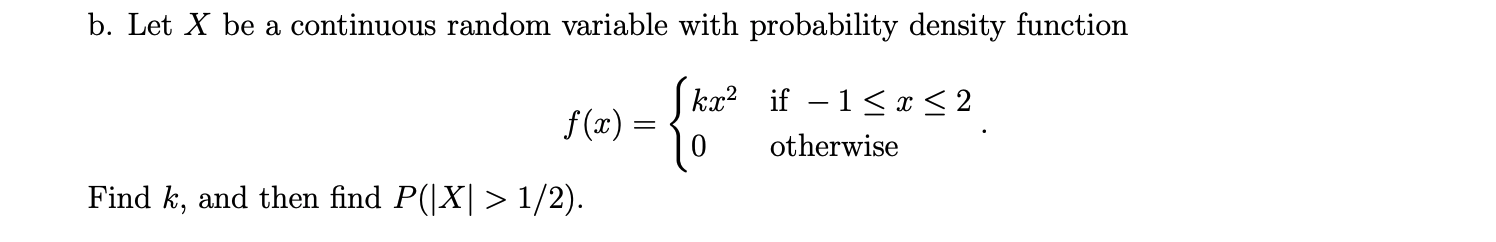b. Let X be a continuous random variable with probability density function f(x) = kx2 if – 1 < x < 2 ) otherwise Find k, and then find P(|X| > 1/2).

• ### Let x be a continuous random variable over [a,b] with probability density function f. Then the...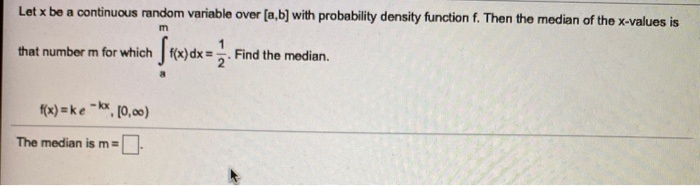Let x be a continuous random variable over [a,b] with probability density function f. Then the median of the x-values is m that number m for which f(x) dx = Find the median. f(x)=ke-kx e-10,00) The median is m=

• ### 3) The continuous random variable X has the probability density function, ), 2 3x3 f(x) =...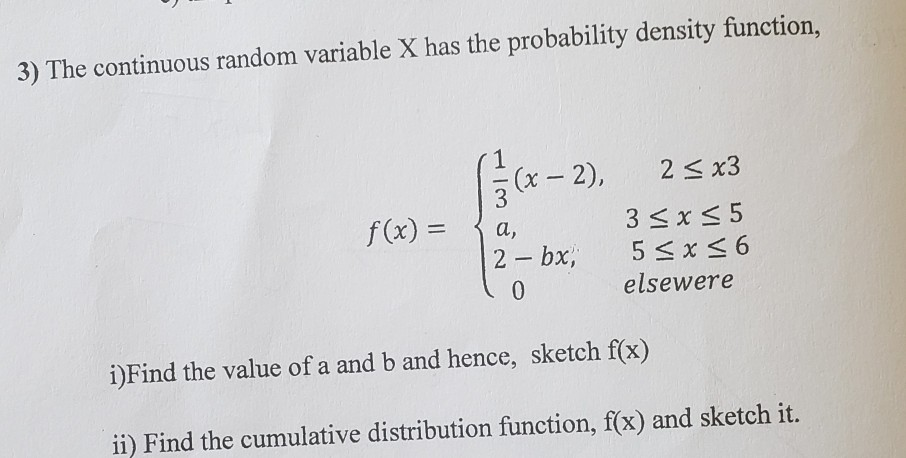3) The continuous random variable X has the probability density function, ), 2 3x3 f(x) = { a, 35x55 2 - bx, 5 < x < 6 elsewere 10 i)Find the value of a and b and hence, sketch f(x) ii) Find the cumulative distribution function, f(x) and sketch it.

• ### 2. Suppose X is a continuous random variable with the probability density function (i.e., pdf) given...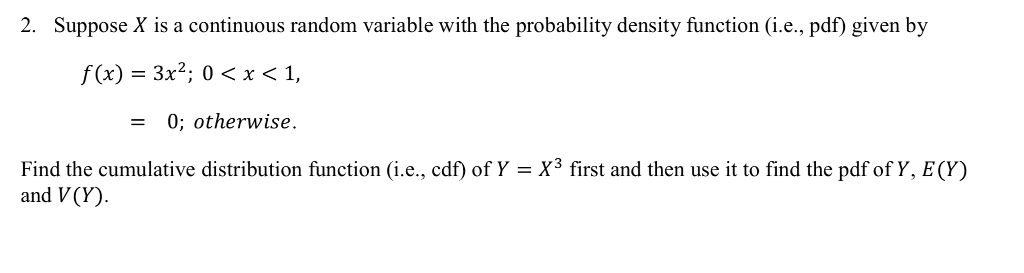2. Suppose X is a continuous random variable with the probability density function (i.e., pdf) given by f(x) - 3x2; 0< x < 1, - 0; otherwise Find the cumulative distribution function (i.e., cdf) of Y = X3 first and then use it to find the pdf of Y, E(Y) and V(Y)

• ### Let X be a continuous random variable with the following probability density function f 0 <...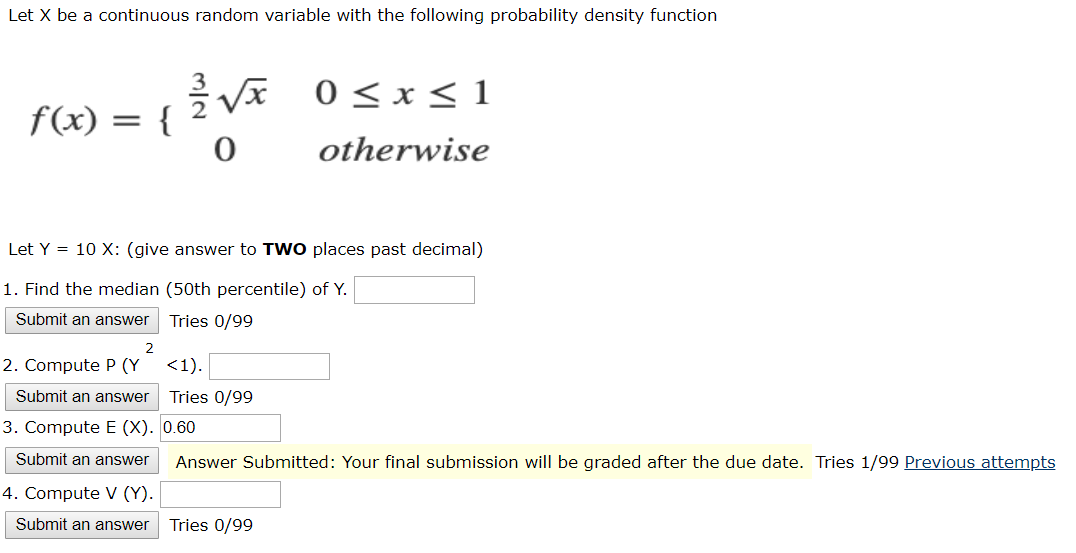Let X be a continuous random variable with the following probability density function f 0 < x < 1 otherwise 0 Let Y = 10 X: (give answer to two places past decimal) 1. Find the median (50th percentile) of Y. Submit an answer Tries 0/99 2. Compute p (Y' <1). Submit an answer Tries 0/99 3. Compute E (X). 0.60 Submit an answer Answer Submitted: Your final submission will be graded after the due date. Tries 1/99 Previous attempts...

• ### (1) Suppose that X is a continuous random variable with probability density function 0<x< 1 f()...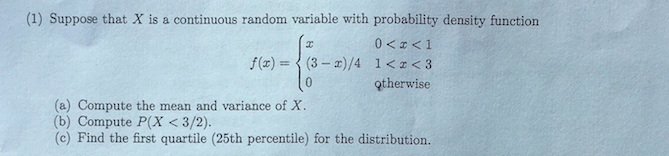(1) Suppose that X is a continuous random variable with probability density function 0<x< 1 f() = (3-X)/4 i<< <3 10 otherwise (a) Compute the mean and variance of X. (b) Compute P(X <3/2). (c) Find the first quartile (25th percentile) for the distribution.

• ### 2. The random variable X has probability density function f given by f(x) 0 otherwise. (a)...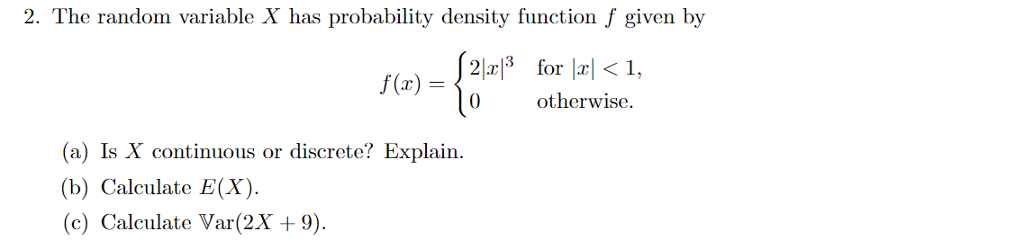2. The random variable X has probability density function f given by f(x) 0 otherwise. (a) Is X continuous or discrete? Explain. (b) Calculate E(X). (c) Calculate Var(2X 9).